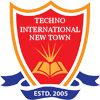# Communication Engg. Lab

COMMUNICATION ENGG. AND CODING THEORY LABORATORY

Code: CS-491

List of Experiments:
1. Measurement of modulation index of AM signals.
2. Demodulation of AM signal.
3. Generation of FM using VCO.
4. Demodulation of FM signal.
5. Generation of PAM.
6. Generation of PWM & PPM.
7. Study of PLL.
8. An Innovative Experiment.

DIGITAL COMMUNICATION LABORATORY

Code: EC691

List of Experiments:

1. Design, implementation and study of all the properties of 7-length and 15-length pn sequences using shift register.

2. Study of PAM and demodulation.

3. Study of PCM and demodulation.

4. Study of line coders: polar/unipolar/bipolar NRZ, RZ and Manchester.

5. Study of delta modulator and demodulator.

6. Study of adaptive delta modulator and demodulator.

7. Study of BPSK modulator and demodulator.

8. Study of BFSK modulator and demodulator.

9. Study of ASK modulator and demodulator.

10. Study of QPSK modulator and demodulator.

11. Simulation study of probability of symbol error for BPSK modulation.

12. Simulation study of probability of symbol error for BFSK modulation.

ANALOG COMMUNICATION LABORATORY

Code: EC 591

List of Experiments:

1. Measurement of modulation index of AM signals.
2. Measurement of selectivity, sensitivity, fidelity of an AM superheterodyne receiver.
3. Demodulation of AM signal.
4. Study of frequency modulation
5. Spectrum analysis of FM signal.
6. Demodulation of FM signal.
7. Study of VCO.
8. Study of PLL.
9. An Innovative Experiment